# A newspaper's

A newspaper's cover page is 3/8 text, and photographs fill the rest. If 2/5 of the text is an article about endangered species, what fraction of the whole cover page is the article about endangered species?

x =  0.15

### Step-by-step explanation:Did you find an error or inaccuracy? Feel free to write us. Thank you!Tips to related online calculators
Need help to calculate sum, simplify or multiply fractions? Try our fraction calculator.

## Related math problems and questions:

• Pizza cover5/8 of the pizza is covered in mushrooms, 1/4 of the pizza is covered with pineapples, and the rest of the pizza is covered with only cheese. What fraction of the pizza is covered with only cheese?
• Cake fractions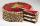Thomas ate 1/3 of cake, Bohouš of the rest of the cake ate 2/5. What fraction of cake left over for others?
• SarahSarah has a photography blog. 3/7 of her photos are of nature, 1/4 of the rest are of her friends. What fraction of all of Sarah's photos is of her friends?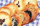In a cookie jar, 1/4 of the cookies are chocolate chip and 1/2 of the rest are peanut butter. What fraction of all the cookies are peanut butter?
• Equivalent fractions 2Write the equivalent multiplication expression. 2 1/6÷3/4
• Product of two fractionsProduct of two fractions is 9 3/5 . If one of the fraction is 9 3/7. Find the other fraction.
• FractionFind for what x fraction (-4x -6)/(x) equals:
• Living room 3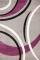Pearl wants to buy carpet to cover her whole living room, except for the tiled floor. The tiled floor is 7 1/2 ft by 3 1/4 ft. Find the area the carpet needs to cover.
• CompareCompare fractions (34)/(3) and (12)/(4). Which fraction of the lower?
• Human 3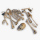Human bones are 3/10 living tissues 9/20 minerals, and the rest water. What fraction of human bone is water?
• The BakeryAt a Bakery, ⅗ of the baked goods are pies, and the rest are cakes. ⅓ of the pies are coconut. ⅙ of the cakes are angel food. What fraction of all the baked goods at the Bakery are coconut pies? What fraction of all the baked goods at the Bakery are are a
• Write 3Write a real-world problem involving the multiplication of a fraction and a whole number with a product that is between 8 and 10, then solve the problem.
• What fraction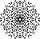What fraction of number 1 to 30 is prime?
• Sign of a expressionWhat is the sign of expression: minus 18 start fraction 9 divided by 17 end fraction plus left parenthesis minus 18 start fraction 9 divided by 17 end fraction right parenthesis?
• Mixed numbersRewrite mixed numbers, so the fractions have the same denominator: 5 1/5 - 2 2/3
• Division by zero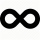Fraction 5 by 2. if 3 is added to numerator and 2 is subtracted from the denominator then the new fraction is:
• Self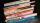A math text book is 2 2/9 inches thick. how many of these books will fit on a 120-inch self?